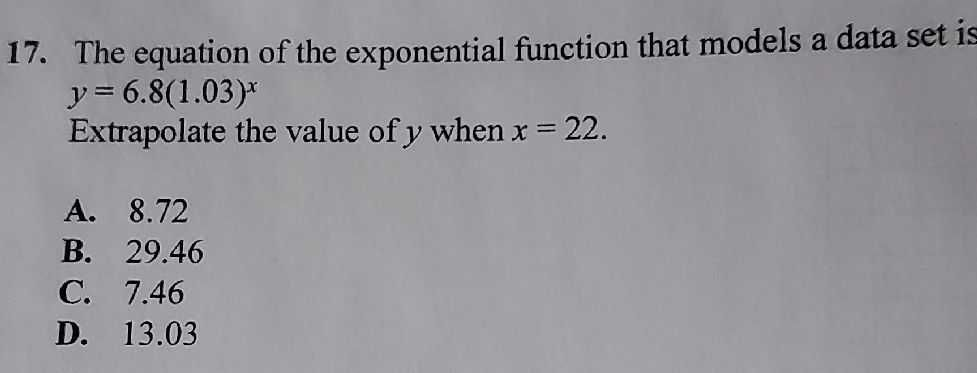### ¿Todavía tienes preguntas de matemáticas?

Pregunte a nuestros tutores expertos
Algebra
Pregunta17. The equation of the exponential function that models a data set is $$y = 6.8 ( 1.03 ) ^ { x }$$

Extrapolate the value of $$y$$ when $$x = 22$$ . A. $$8.72$$ B. $$29.46$$ C. $$7.46$$ D. $$13.03$$